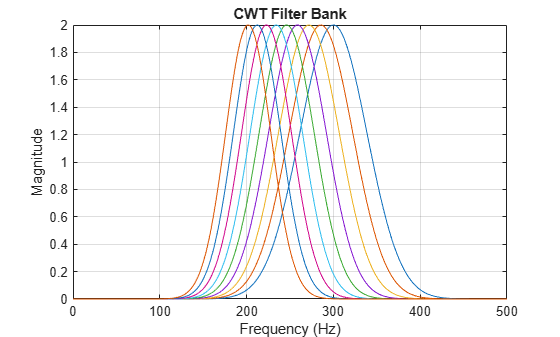Main Content

# freqz

CWT filter bank frequency responses

## Syntax

``[psidft,f] = freqz(fb)``
``freqz(fb)``

## Description

example

````[psidft,f] = freqz(fb)` returns the frequency responses for the wavelet filters, `psidft`, and the frequency vector, `f`, for the continuous wavelet transform (CWT) filter bank, `fb`. Frequencies are in cycles/sample or Hz. If you specify a sampling period, the frequencies are in cycles/unit time where the time unit is the unit of the duration `SamplingPeriod`.The frequency responses, `psidft`, are one-sided frequency responses for the positive frequencies. For the analytic wavelets supported by `cwtfilterbank`, the frequency responses are real-valued and are equivalent to the magnitude frequency response.```
````freqz(fb)` with no output arguments plots the magnitude frequency responses for the CWT filter bank, `fb`.```

## Examples

collapse all

Create a CWT filter bank. Set the voices per octave to 14, the sampling frequency to 1000 Hz, and frequency limits to range from 200 Hz to 300 Hz.

`fb = cwtfilterbank('VoicesPerOctave',14,'SamplingFrequency',1000,'FrequencyLimits',[200 300])`
```fb = cwtfilterbank with properties: VoicesPerOctave: 14 Wavelet: 'morse' SamplingFrequency: 1000 SamplingPeriod: [] PeriodLimits: [] SignalLength: 1024 FrequencyLimits: [200 300] TimeBandwidth: 60 WaveletParameters: [] Boundary: 'reflection' ```

Obtain the frequency responses for the filter bank. Plot the frequency responses.

```[psidft,f] = freqz(fb); plot(f,psidft) grid on title('Frequency Responses') xlabel('Frequency (Hz)') ylabel('Magnitude')```## Input Arguments

collapse all

Continuous wavelet transform (CWT) filter bank, specified as a `cwtfilterbank` object.

## Output Arguments

collapse all

Frequency responses of a CWT filter bank, returned as a real-valued `Ns`-by-`M` matrix where `Ns` is the number of scales. If the filter bank `Boundary` is `'reflection'`, `M` is equal to `2*floor(SignalLength/2)+1`. If the filter bank `Boundary` is `'periodic'`, `M` is equal to `floor(SignalLength/2)+1`.

The frequency responses, `psidft`, are one-sided frequency responses for the positive frequencies. For the analytic wavelets supported by `cwtfilterbank`, the frequency responses are real-valued and are equivalent to the magnitude frequency response.

Frequencies, in cycles/sample or Hz, returned as a real-valued vector. If the filter bank `Boundary` is `'reflection'`, `f` has length `2*floor(SignalLength/2)+1`. If the filter bank `Boundary` is `'periodic'`, `f` has length `floor(SignalLength/2)+1`.

If you specify a sampling period, the frequencies are in cycles/unit time where the time unit is the unit of the duration `SamplingPeriod`.

Data Types: `double`

## See Also

Introduced in R2018a

## SupportGet trial now